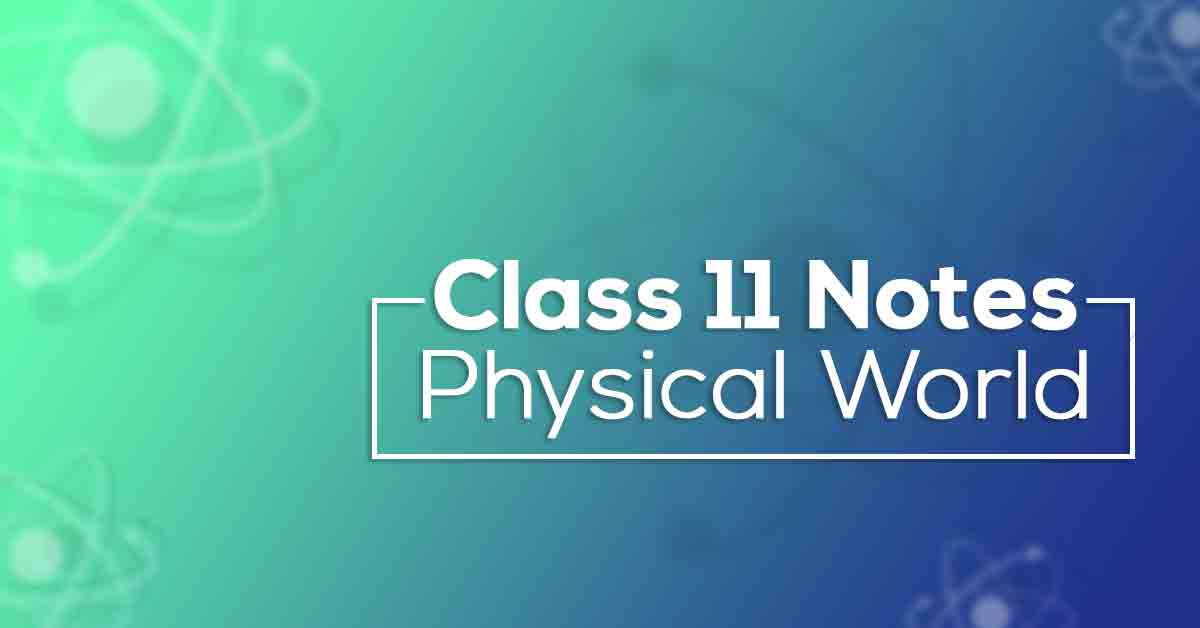Class 11th Physics Physical World NCERT Notes CBSE 2023

# Class 11 Physics Chapter 1 Physical World Notes# Chapter 1 Physical World

Chapter 1 of Year 11 Physics is called "The Physical World". Students learn basic concepts like physics, their role and importance in everyday life and the world around them. Chapter 1, The Physical World, will also help students build a solid foundation for more advanced topics to explore further. NCERT notes Class 11 Physics Chapter 1 will help students answer questions.

This chapter deals specifically with physics, the scope and chaos of physics, technology and society, the fundamental forces of nature and the nature of the laws of physics. Students should practice all text questions provided by Vidyakul. Read on to learn about the NCERT notes to Class 11 Physics.

### Points to Remember

Below, we have mentioned some of the important points to remember from NCERT Class 11 Physics chapter 1.

Physics is a branch of science which explains nature and natural phenomena.

• The basic laws of physics are universal and apply in widely different contexts and conditions.
• Branches of Classical physics are Mechanics, Thermodynamics, Electromagnetism, Optics and Acoustics.
• Branches of Modern Physics are Relativity, Quantum Mechanics, Atomic and Nuclear Physics.
• Physics and technology are related to each other. Sometimes technology gives rise to new physics; at other times, physics generates new technology. Both have a direct impact on society.
• Gravitational force: It is the force of mutual attraction between any two objects by virtue of their masses. It is a universal force.
• Electromagnetic force: It is the force between charged particles. When charges are at rest, the force between changes is given by Coulombs law. Charges in motion produce magnetic effects and magnetic field, which apply force on moving charges. Electric and magnetic effects are inseparable, hence the name is given as electromagnetic force. Electromagnetic forces are very strong compared to gravitational forces.
• Strong nuclear force: Strong nuclear force binds protons and neutrons in a nucleus. The strong nuclear force is the strongest of all fundamental forces, about 100100 times the electromagnetic force in strength.

Weak nuclear force: The weak nuclear force appears only in certain nuclear processes such as the ββ-decay of a nucleus. The weak nuclear force is not as weak as the gravitational force, but much weaker than the strong nuclear and electromagnetic forces.

### Topics and Sub-topics

Chapter 1 of Physics for Grade 11 may seem difficult to understand at first. However, students who study the concept videos before practicing the problems can easily solve them. Students should practice solving all kinds of questions so that they can solve all kinds of questions asked on the exam. This allows students to understand the nature of the question and analyze the question types.

Vidyakul provides NCERT notes to answer all your CBSE Physics Class 11 Chapter 1 Physics textbook questions based on the latest curriculum. Vidyakul's questions are provided free of charge to help students score well on their exams.

Below, we have provided the list of topics included in this chapter:

 S.No Topic Name 1 Understanding Physics 2 Scope of Physics 3 Relation between Physics, Technology and Society 4 Fundamental Forces of Nature 5 Nature of Physical Laws

### Few Important Questions

• Who found the subject ‘Physics’?

Galileo has been called the “father of modern observational astronomy”.

• What are the uses of the ‘physics’ subject?

Physics is used heavily in engineering. For example, statics, a subfield of mechanics, is used in the building of bridges and other static structures.

• What are the fundamental of physics?

Energy Transfer Model (ETM)2. Universal Gravitation (escape velocity, etc) (CFPM)3. Simple Harmonic Motion (OPM)

### Practice Questions

What do you think did Einstein mean when he said: “The most incomprehensible thing about the world is that it is comprehensible”?

“Every great physical theory starts as a heresy and ends as a dogma”. Give some examples from the history of the science of the validity of this incisive remark.

“Politics is the art of the possible”. Similarly, “Science is the art of the soluble”. Explain this aphorism on the nature and practice of science.